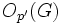# Glauberman type for a prime divisor implies not simple non-abelian

## Statement

Suppose$G$ is a finite group and$p$ is a prime number dividing the order of$G$. Suppose further that$G$ is a group of Glauberman type for$p$. Then,$G$ is not a simple non-abelian group.

## Proof

Given: A group$G$, a prime$p$ dividing the order of$G$ such that$O_{p'}(G)N_G(Z(J(P)) = G$.

To prove:$G$ is not simple non-abelian.

Proof: We consider two cases:

•$O_{p'}(G)$ is nontrivial: In this case,$O_{p'}(G)$ is a proper nontrivial normal subgroup of$G$. It is proper because$p$ divides the order of$G$.
•$O_{p'}(G)$ is trivial: In this case,$N_G(Z(J(P))) = G$. Thus,$Z(J(P))$ is normal in$G$. Since$p$ divides the order of$G$,$P$ is nontrivial, hence, since the ZJ-functor, by definition, sends nontrivial$p$-subgroups to nontrivial$p$-subgroups,$Z(J(P))$ is nontrivial. Thus,$Z(J(P))$ is a nontrivial normal subgroup of$G$. The only way it can be the whole group is if$P$ is abelian. Hence, we either have a proper nontrivial normal subgroup or have that$P$ is abelian. In either case, we are done.